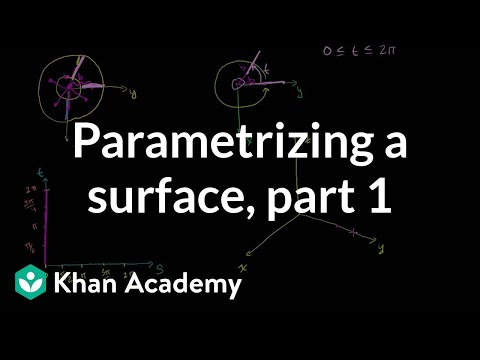### Calculus: Surface integrals and Stokes' theorem

Popular Free Closed [?]Parameterizing a surface. Surface integrals. Stokes' theorem.
Introduction to Parametrizing a Surface with Two Parameters. Determining a Position Vector-Valued Function for a Parametrization of Two Parameters. Partial Derivatives of Vector-Valued Functions. Introduction to the Surface Integral. Example of calculating a surface integral part 1. Example of calculating a surface integral part 2. Example of calculating a surface integral part 3. Surface Integral Example Part 1 - Parameterizing the Unit Sphere. Surface Integral Example Part 2 - Calculating the Surface Differential. Surface Integral Example Part 3 - The Home Stretch. Surface Integral Ex2 part 1 - Parameterizing the Surface. Surface Integral Ex2 part 2 - Evaluating Integral. Surface Integral Ex3 part 1 - Parameterizing the Outside Surface. Surface Integral Ex3 part 2 - Evaluating the Outside Surface. Surface Integral Ex3 part 3 - Top surface. Surface Integral Ex3 part 4 - Home Stretch. Conceptual Understanding of Flux in Three Dimensions. Constructing a unit normal vector to a surface. Vector representation of a Surface Integral. Stokes' Theorem Intuition. Green's and Stokes' Theorem Relationship. Orienting Boundary with Surface. Orientation and Stokes. Conditions for Stokes Theorem. Stokes Example Part 1. Part 2 Parameterizing the Surface. Stokes Example Part 3 - Surface to Double Integral. Stokes Example Part 4 - Curl and Final Answer. Evaluating Line Integral Directly - Part 1. Evaluating Line Integral Directly - Part 2. Stokes' Theorem Proof Part 1. Stokes' Theorem Proof Part 2. Stokes' Theorem Proof Part 3. Stokes' Theorem Proof Part 4. Stokes' Theorem Proof Part 5. Stokes' Theorem Proof Part 6. Stokes' Theorem Proof Part 7. Introduction to Parametrizing a Surface with Two Parameters. Determining a Position Vector-Valued Function for a Parametrization of Two Parameters. Partial Derivatives of Vector-Valued Functions. Introduction to the Surface Integral. Example of calculating a surface integral part 1. Example of calculating a surface integral part 2. Example of calculating a surface integral part 3. Surface Integral Example Part 1 - Parameterizing the Unit Sphere. Surface Integral Example Part 2 - Calculating the Surface Differential. Surface Integral Example Part 3 - The Home Stretch. Surface Integral Ex2 part 1 - Parameterizing the Surface. Surface Integral Ex2 part 2 - Evaluating Integral. Surface Integral Ex3 part 1 - Parameterizing the Outside Surface. Surface Integral Ex3 part 2 - Evaluating the Outside Surface. Surface Integral Ex3 part 3 - Top surface. Surface Integral Ex3 part 4 - Home Stretch. Conceptual Understanding of Flux in Three Dimensions. Constructing a unit normal vector to a surface. Vector representation of a Surface Integral. Stokes' Theorem Intuition. Green's and Stokes' Theorem Relationship. Orienting Boundary with Surface. Orientation and Stokes. Conditions for Stokes Theorem. Stokes Example Part 1. Part 2 Parameterizing the Surface. Stokes Example Part 3 - Surface to Double Integral. Stokes Example Part 4 - Curl and Final Answer. Evaluating Line Integral Directly - Part 1. Evaluating Line Integral Directly - Part 2. Stokes' Theorem Proof Part 1. Stokes' Theorem Proof Part 2. Stokes' Theorem Proof Part 3. Stokes' Theorem Proof Part 4. Stokes' Theorem Proof Part 5. Stokes' Theorem Proof Part 6. Stokes' Theorem Proof Part 7.

Categories:

# Reviews

-- no reviews yet -- make the first review

# Alternatives All alternatives to all Khan Academy courses

-- no alternatives found for the course --
If you know any alternatives, please let us know.

# Prerequisites

-- no prerequsites found for the course --
If you can suggest any prerequisite, please let us know.

# Paths

No Paths inclusing the course. You can build and share a path with this course included.

# Certification Exams

-- there are no exams to get certification after this course --
If your company does certification for those who completed this course then register your company as certification vendor and add your exams to the Exams Directory.

# Similar courses

Courses related to the course subject

### Calculus I (Differential Calculus)

Videos on a first course in calculus (Differential Calculus).

### Calculus II (Integral Calculus)

Videos on a second course in calculus (Integral Calculus).

### Calculus One

Calculus One is a first introduction to differential and integral calculus, emphasizing engaging examples from everyday life.

Details:

### Calculus: AP Calculus practice questions

Sample questions from the A.P. Calculus AB and BC exams (both multiple choice and free answer). 2011 Calculus AB Free Response #1a…

### Calculus: Derivative applications

Minima, maxima, and critical points. Rates of change. Optimization. Rates of change. L'Hopital's rule. Mean value theo…

### Calculus: Divergence theorem

Divergence theorem intuition. Divergence theorem examples and proofs. Types of regions in 3D. 3-D Divergence Theorem Intuition.

### Calculus: Double and triple integrals

Volume under a surface with double integrals. Triple integrals as well. Double Integral 1. Double Integrals 2. Double Integrals 3.

### Calculus: Indefinite and definite integrals

Indefinite integral as anti-derivative. Definite integral as area under a curve. Integration by parts. U-substitution. Trig su…

### Calculus: Limits

Limit introduction, squeeze theorem, and epsilon-delta definition of limits. Introduction to limits. Limit at a point of discontin…

### Calculus: Line integrals and Green's theorem

Line integral of scalar and vector-valued functions. Green's theorem and 2-D divergence theorem. Introduction to the Line In…

Let us know when you did the course Calculus: Surface integrals and Stokes' theorem.

 Started on: Completed on: Your grade (if any):Comments:Add the course Calculus: Surface integrals and Stokes' theorem to My Personal Education Path.

 Start the course on: Duration of study: 1 Week 2 Weeks 3 Weeks 4 Weeks 5 Weeks 6 Weeks 7 Weeks 8 Weeks 10 Weeks 12 Weeks 16 Weeks Notes: